# Why do the exterior angles sum up to 360 degrees?

• Module 0 Week 4 Day 13 Challenge Part 4

The topic says everything!

• @RZ923 Thanks for asking! This is a really fun question because the proof is completely visual, in your head. I'm going to attempt to manifest this visualization into something tangible. How about start with an arbitrary polygon, like a septagon?

Our septagon has 7 different colors of angles because we are going to "move" the angles around. Or, in math-speak, "translate" them.The vertices (corners) of each angle are all different; they don't lie on the same point. Here you can see each angle, highlighted separately.# Method 1

We're going to move copies of our septagon so that the highlighted angles "stack" on top of each other: so that their vertices match up. Let's stack them at the corner which houses our $$\textcolor{purple}{\text{purple}}$$ angle.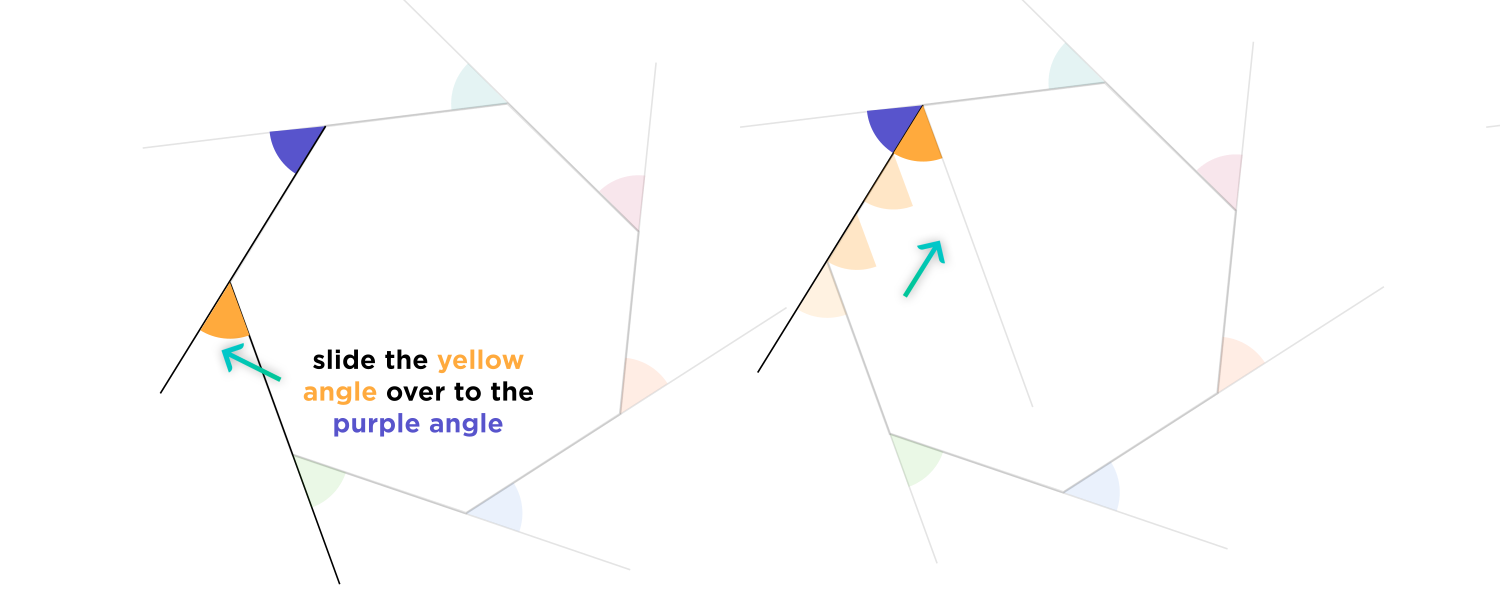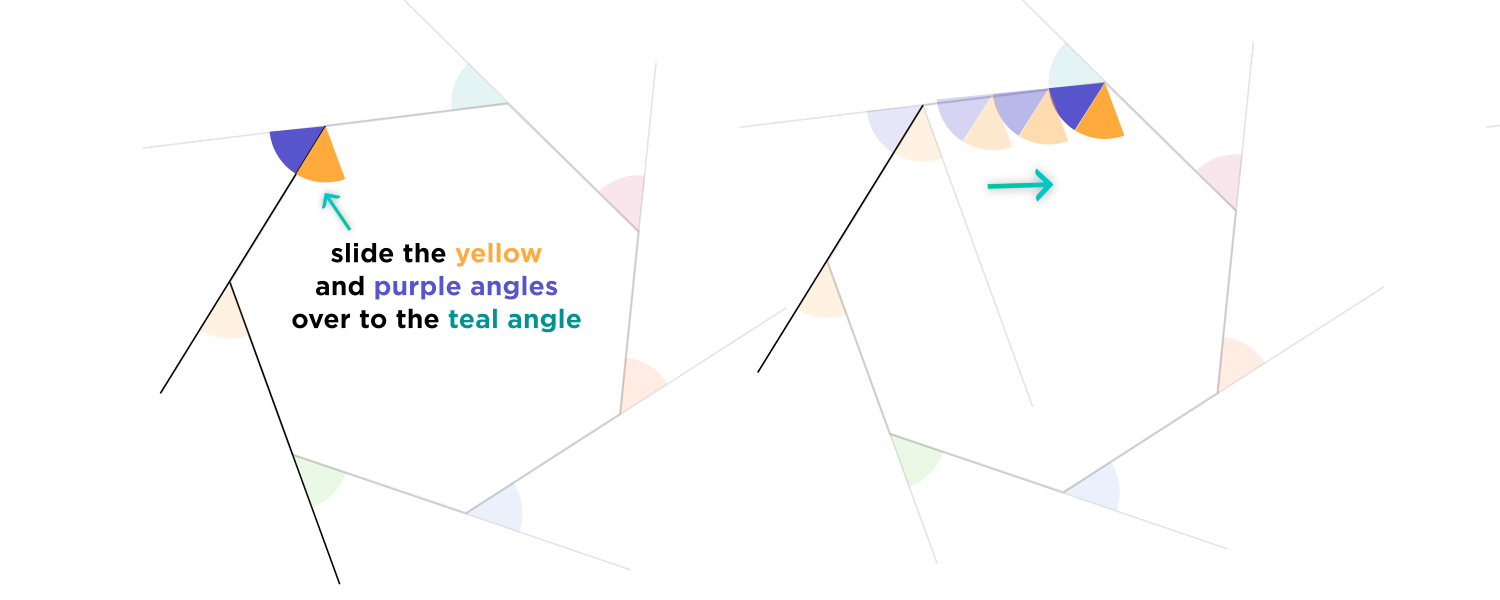It's quite pretty!Does this work if the polygon is not regular (doesn't have sides the same length)? Yes, it does!

Here is a non-regular hexagon:We can slide the angles over in the same way, to show that they add up to $$360^{\circ}.$$# Method 2

This is one way to "see" it inside your head. Another way to prove that the sum of the exterior angles of a polygon is $$360^{\circ}$$ is to use a different method: to chop up the septagon into triangles.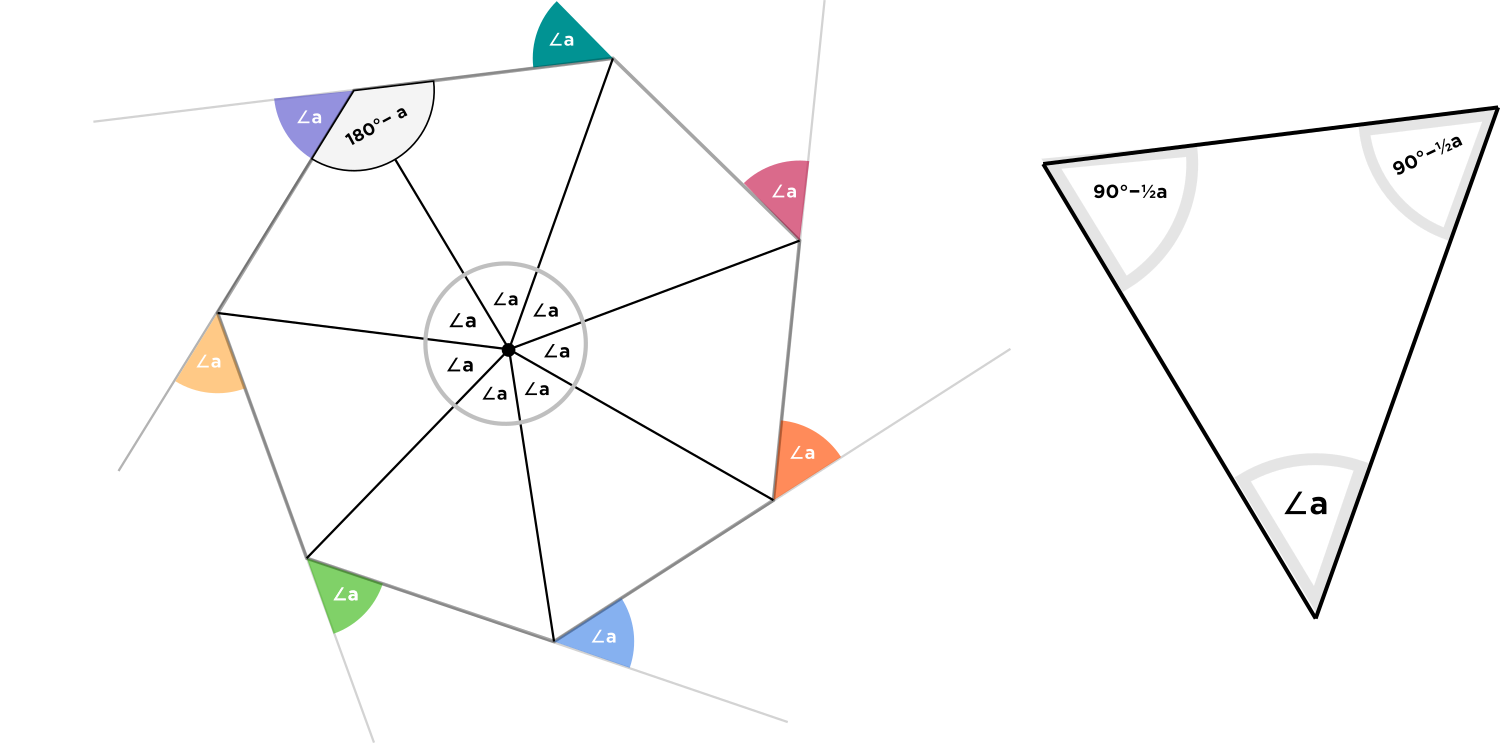The angle $$\angle a$$ found by drawing all the lines which emanate from the center to the corners of the septagon is equal to $$\frac{360^{\circ}}{7}.$$ Each of these triangles is isosceles, so its other two angles are each $$90^{\circ} - \frac{1}{2} \angle a.$$ Two of these angles make an interior angle of the septagon, so an $$\color{red}{\text{ interior angle }}$$ measures $$90^{\circ}-\frac{1}{2}a + 90^{\circ} - \frac{1}{2}a = \color{red}180^{\circ} - a.$$

Since each colored exterior angle adds with an interior angle to make a line, it is supplementary to the interior angle and so has measure $$\angle a.$$ We know that seven of these angle $$\angle a$$'s form a circle around the center of the septagon, so the colored exterior angles all add up to $$360^{\circ}.$$

Does this work even if the polygon isn't regular? Yes, it does!

# Method 3: The "Bus" Proof

Pretend the perimeter of the polygon is a road, and you're on a bus driving along it. At every vertex, the angle of your direction changes. You drive a loop around the shape and return to the same spot that you started at. So you "turned" by one full revolution, which is $$360^{\circ}!$$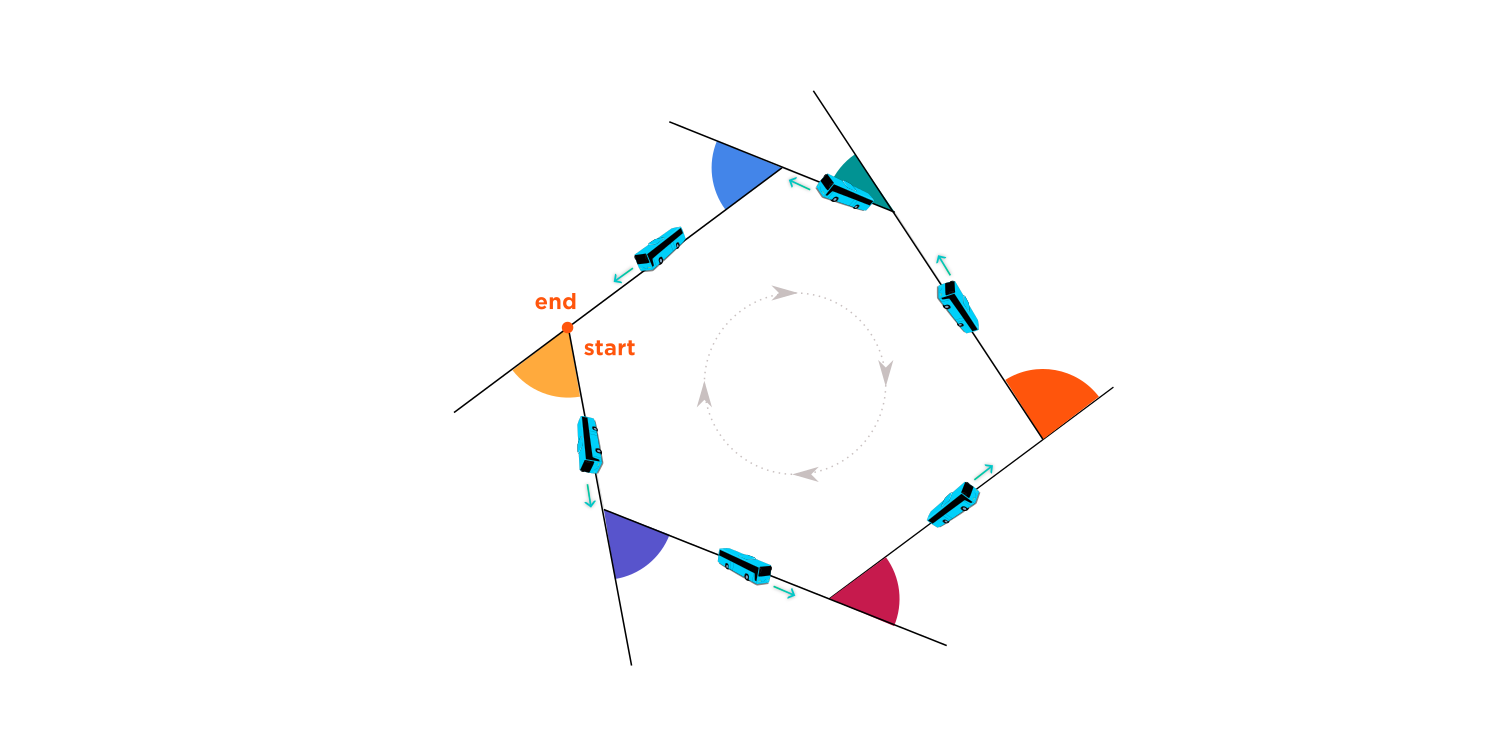As the polygon shrinks smaller and smaller, the angles converge at the center, forming $$360^{\circ}.$$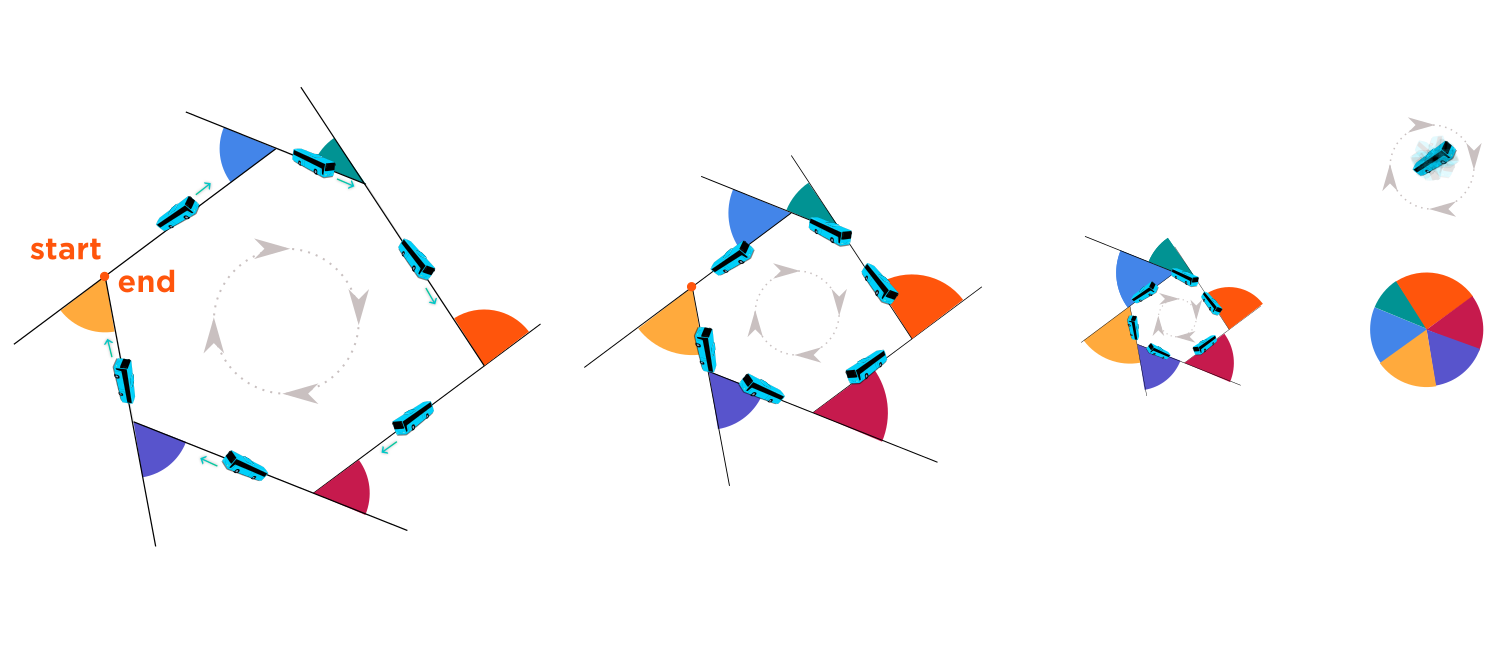Do these proofs work for any polygon with any number of sides? Yes, they do! If the polygon had more sides, there would be more colored angles, on average smaller than the ones shown above. They would still add to $$360^{\circ}.$$

Which proof of the Sum of Exterior Angles of a Polygon is your favorite?• @debbie Thanks